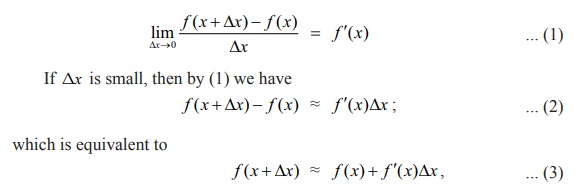Home | | Maths 12th Std | Linear Approximation

# Linear Approximation

In this section, we introduce linear approximation of a function at a point.

Linear Approximation and Differentials

Linear Approximation

In this section, we introduce linear approximation of a function at a point. Using the linear approximation, we shall estimate the function value near a chosen point. Then we shall introduce differential of a real-valued function of one variable, which is also useful in applications.

Let f : (a, b) ŌåÆ ŌäØ be a differentiable function and x Ōłł(a, b) . Since f is differentiable at x , we havewhere Ōēł means ŌĆ£approximatelyŌĆØ equal. Also, observe that as the independent variable changes from x to x + x╬ö, the function value changes from f ( x) to f ( x + ╬öx) . Hence if ╬öx is small and the change in the output is denoted by ╬öf or ╬öy , then (2) can be rewritten as

change in the output = ╬öy = ╬öf = f ( x + ╬öx) ŌłÆ f (x) Ōēł f ŌĆś(x) ╬öx.

Note that (3) helps in approximating the value of f ( x + ╬öx) using f ( x) and f ŌĆś ( x) ╬öx . Also, for a fixed x0 , y( x ) = f ( x0 ) + f ŌĆ▓( x0 )( x ŌłÆ x0 ), x Ōłł R, gives the tangent line for the graph of f at ( x0 , f ( x0 )) which gives a good approximation to the function f near x0 . This leads us to define

Definition 8.1 (Linear Approximation)

Let f : (a, b) ŌåÆ ŌäØ be a differentiable function and x0 Ōłł(a, b) . We define the linear approximation L of f at x0 by

L ( x) = f ( x0 ) + f ŌĆ▓( x0 )( x ŌłÆ x0 ), ŌłĆx Ōłł (a, b)                 ... (4)

Note that by (3) and (4) we see that

f ( x + ╬öx) Ōēł f (x) + f ŌĆ▓ (x) ╬öx ,

which is useful in approximating the value of f ( x + ╬öx).

Note that linear approximation for f at x0 gives a good approximation to f ( x) if x is close to x0 , because

Tangent line x = f (x0) + f ŌĆ▓(x0)(xŌłÆx0)Error = f (x) ŌłÆ L ( x) = f (x ) ŌłÆ f (x0 ) ŌłÆ f ŌĆ▓(x0 )(x ŌłÆ x0 )    ... (5)

approaches zero as x approaches to x0 by continuity of f at x0 . Also, if f ( x) = mx + c , then its linear approximation is L ( x) = (mx0 + c ) + m(x ŌłÆ x0 ) = mx + c = f (x) , for any point x Ōłł(a, b) . That is, the linear approximation, in this case, is the original function itself (is it not surprising?).

Example 8.1

Find the linear approximation for f (x) = ŌłÜ(1+x) , x Ōēź ŌłÆ1, at x0 = 3. Use the linear approximation to estimate f (3.2) .

Solution

We know from (4), that L(x) = f (x0) + f ŌĆ▓(x0)(x ŌłÆ x0) . We have x0 = 3, ╬öx = 0.2 and hence f (3) = ŌłÜ(1+ 3) = 2 . Also,Actually, if we use a calculator to calculate we get ŌłÜ4.2 = 2.04939.

Tags : Mathematics , 12th Maths : UNIT 8 : Differentials and Partial Derivatives
Study Material, Lecturing Notes, Assignment, Reference, Wiki description explanation, brief detail
12th Maths : UNIT 8 : Differentials and Partial Derivatives : Linear Approximation | Mathematics